Math resources Statistics and probability

# Statistics and probability

Here you will find all the statistics and probability resources you need. Like all our math topic guides, we’ve included step-by-step guides, detailed examples and practice questions!Histogram

Histogram step-by-step topic guide, detailed examples, practice questions, teaching tips and FAQs.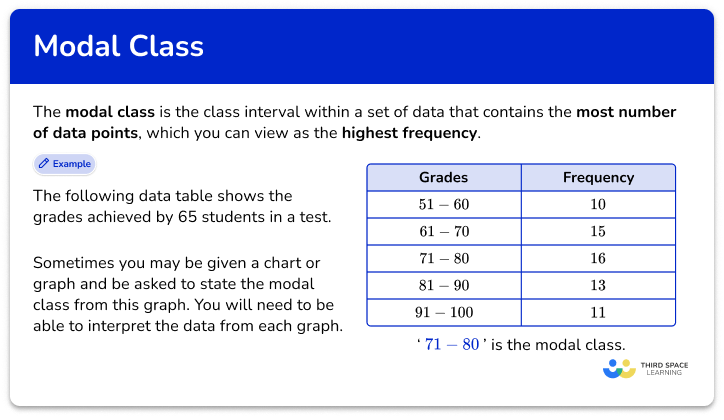Modal class

Modal class step-by-step topic guide, detailed examples, practice questions, teaching tips and FAQs.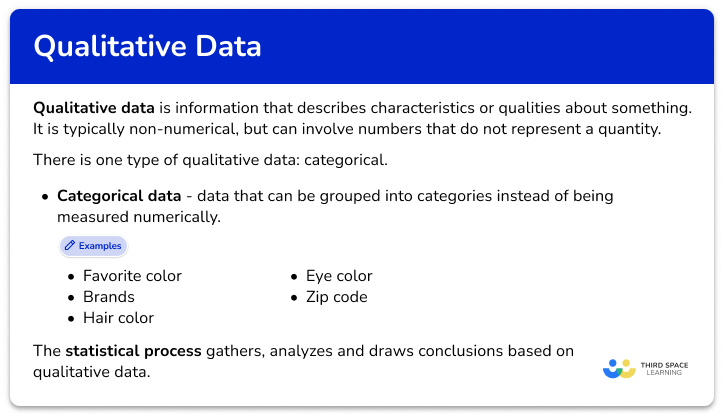Qualitative data

Qualitative data step-by-step topic guide, detailed examples, practice questions, teaching tips and FAQs.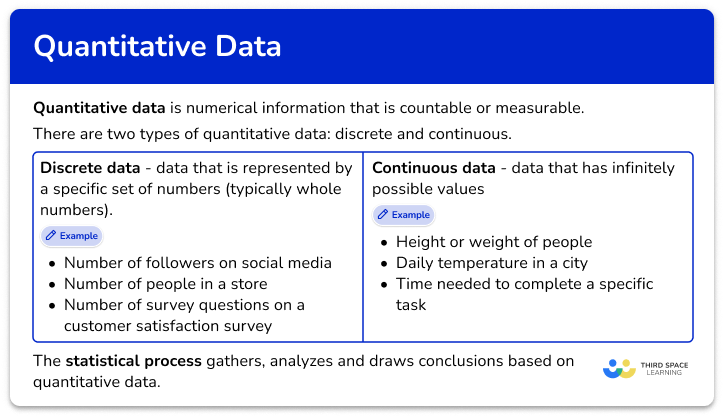Quantitative data

Quantitative data step-by-step topic guide, detailed examples, practice questions, teaching tips and FAQs.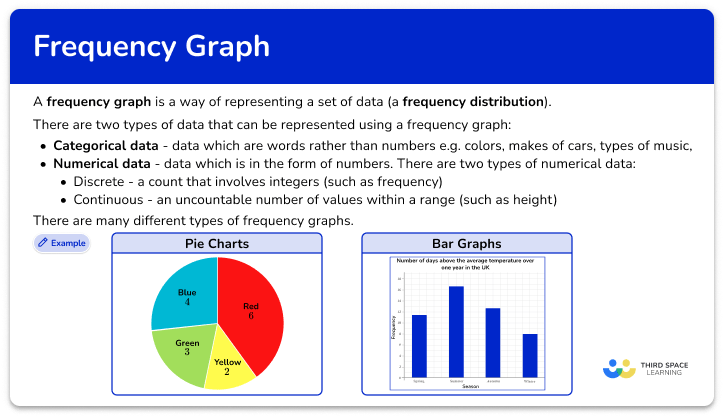Frequency graph

Frequency graph step-by-step topic guide, detailed examples, practice questions, teaching tips and FAQs.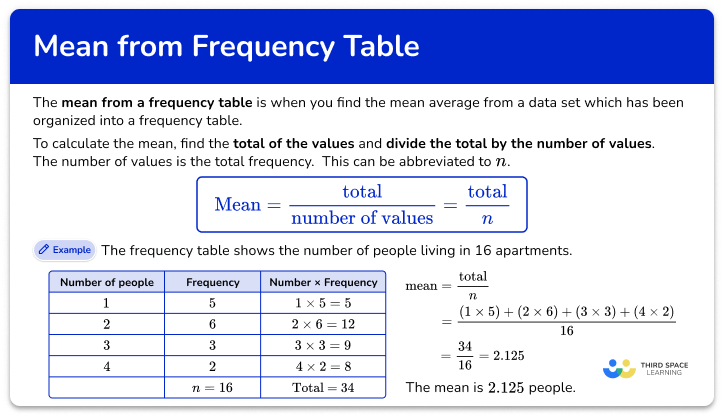Mean from a frequency table

Mean from a frequency table step-by-step topic guide, detailed examples, practice questions, teaching tips and FAQs.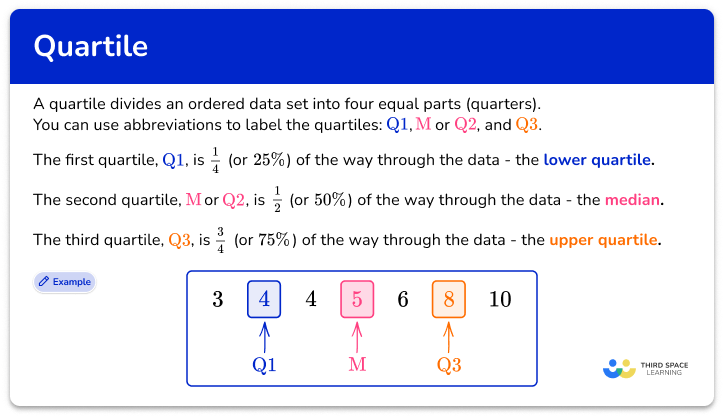Quartile

Quartile step-by-step topic guide, detailed examples, practice questions, teaching tips and FAQs.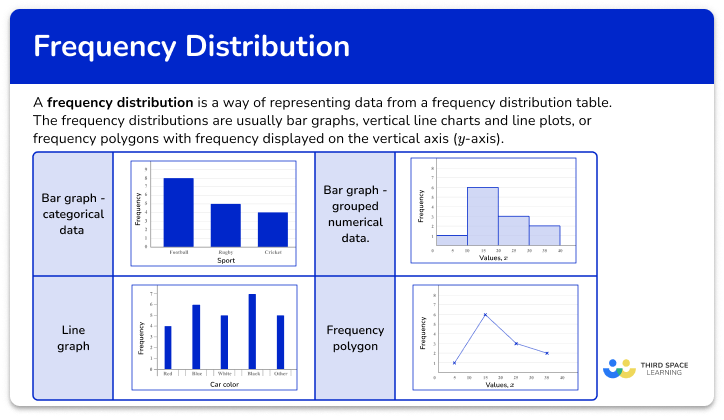Frequency distribution

Frequency distribution step-by-step topic guide, detailed examples, practice questions, teaching tips and FAQs.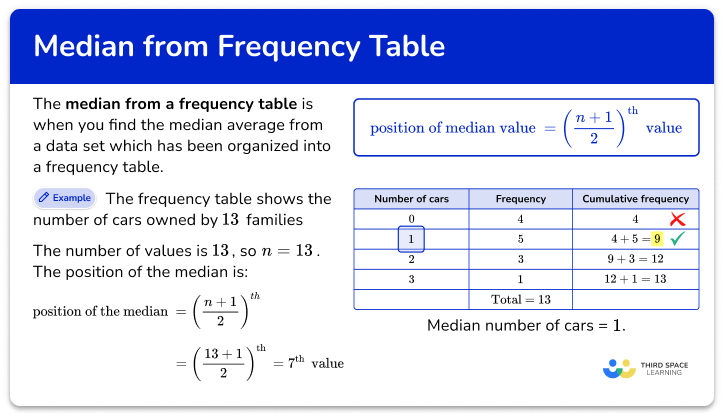How to find the median from a frequency table

How to find the median from a frequency table step-by-step topic guide, detailed examples, practice questions, teaching tips and FAQs.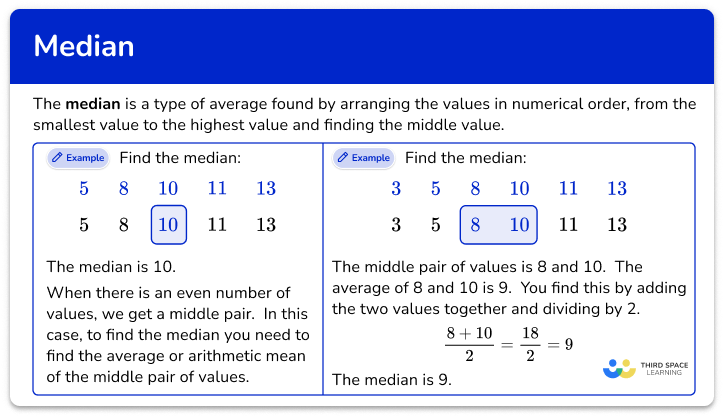Median

Median step-by-step topic guide, detailed examples, practice questions, teaching tips and FAQs.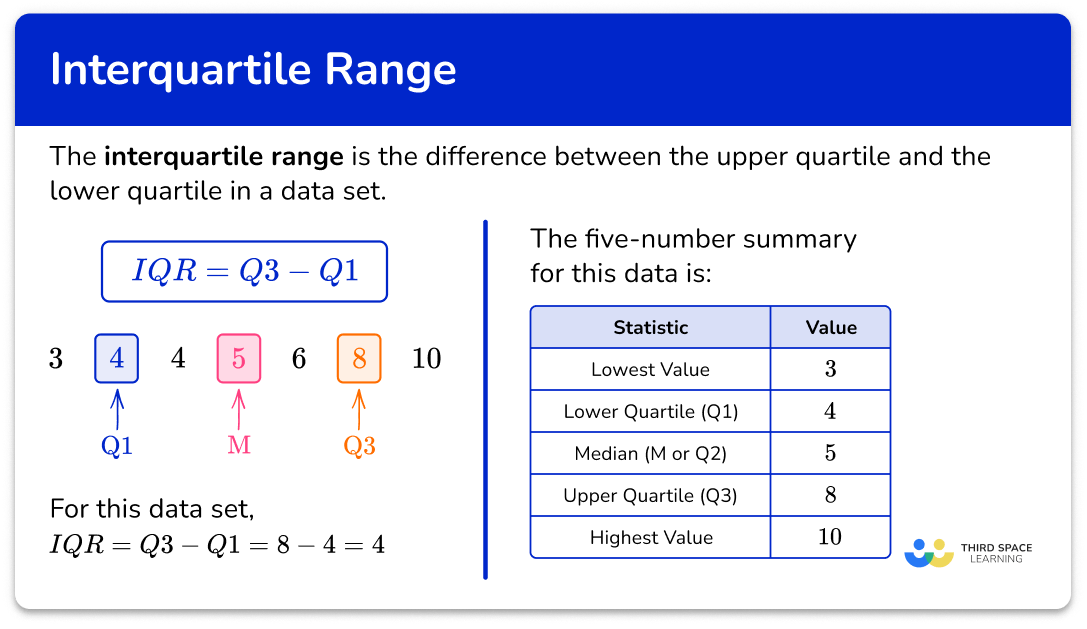Interquartile range

Interquartile range step-by-step topic guide, detailed examples, practice questions, teaching tips and FAQs.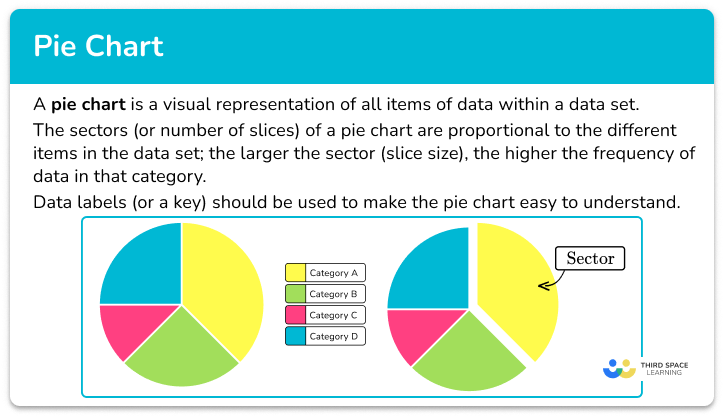Pie chart

Pie chart step-by-step topic guide, detailed examples, practice questions, teaching tips and FAQs.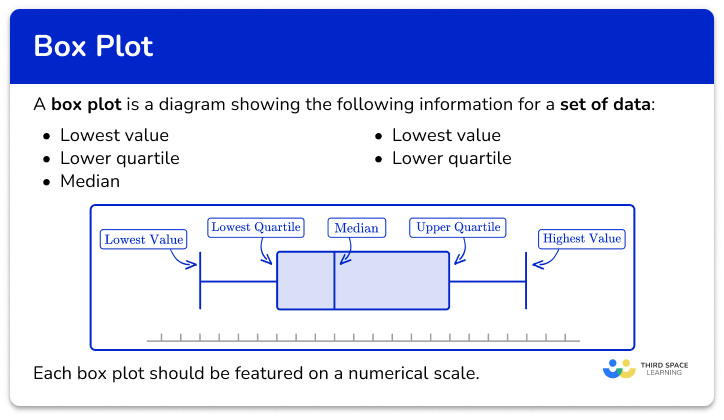Box plot

Box plot step-by-step topic guide, detailed examples, practice questions, teaching tips and FAQs.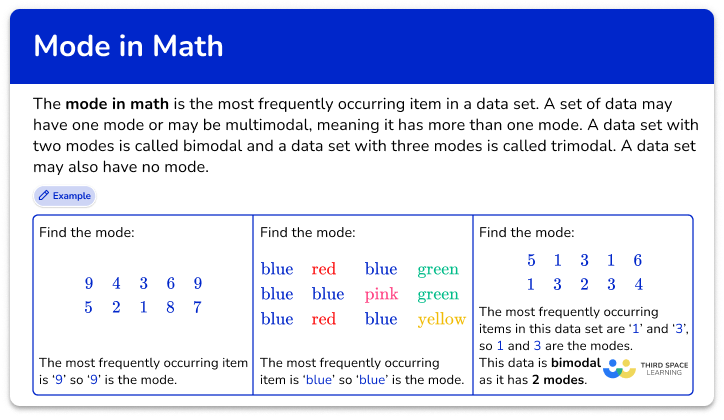Mode in math

Mode in math step-by-step topic guide, detailed examples, practice questions, teaching tips and FAQs.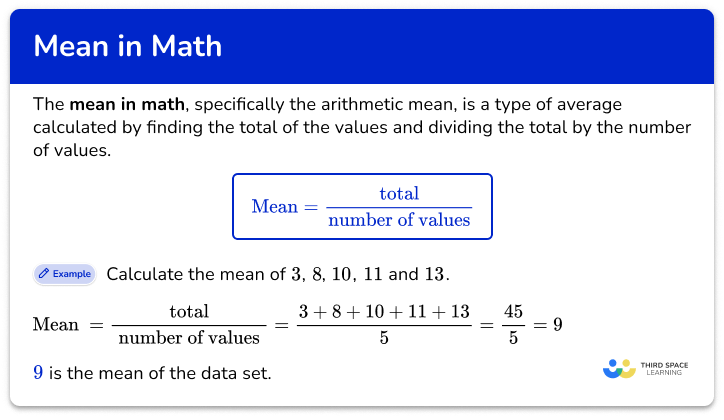Mean in math

Mean in math step-by-step topic guide, detailed examples, practice questions, teaching tips and FAQs.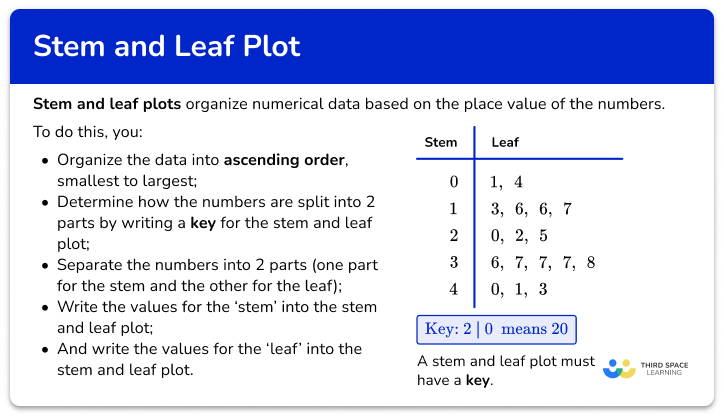Stem and leaf plot

Stem and leaf plot step-by-step topic guide, detailed examples, practice questions, teaching tips and FAQs.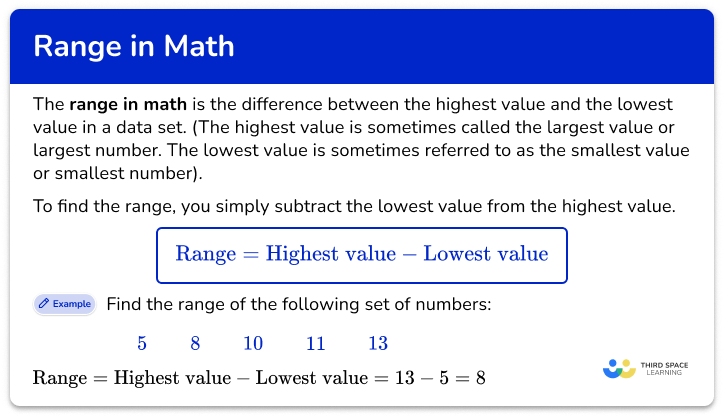Range in math

Range in math step-by-step topic guide, detailed examples, practice questions, teaching tips and FAQs.